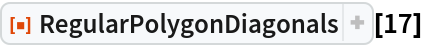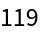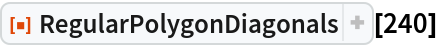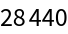#Function Repository Resource:

# RegularPolygonDiagonals

Find the number of diagonals of a regular polygon

Contributed by: Wolfram|Alpha Math Team  |  Wolfram|Alpha Math Team
 ResourceFunction["RegularPolygonDiagonals"][n] returns the number of diagonals of a regular polygon with n sides.

## Examples

### Basic Examples (2)

Find the number of diagonals of a regular polygon with 17 sides:

 In:=Out=Find the number of diagonals of a regular polygon with 240 sides:

 In:=Out=